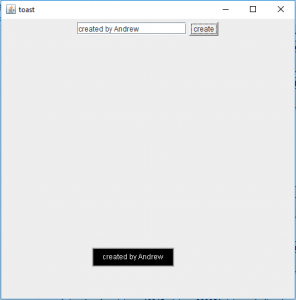# Java Swing | Creating a Toast Message

What are toast messages? And how to create them by using Java Swing?
Toast Messages are a quick way of informing the user by short Pop-up messages that last for a short period of time and then disappear.

Java Swing does not have an inbuilt class for toast message but toast message is a popular and an effective way to display auto-expiring message that is displayed only for a short length of time. So in order to implement a toast message we have to manually build a class that is capable of creating a toast message.

In this article we will discuss how to manually create a toast message in Java, using Java Swing Components. The Following programs will create a text toast message that lasts for a short length of time and then they will disappear.

Please go through the following article for more details on translucent Window and Frames, This will give you the idea how to implement translucent and shaped window.
Java Swing | Translucent and Shaped Window in Java
JSwing | Translucent and Shaped window

The following program creates the toast message (which is a selectively translucent JWindow)

 `// Java program that creates the toast message ` `//(which is a selectively translucent JWindow) ` `import` `java.awt.*; ` `import` `javax.swing.*; ` `import` `java.awt.event.*; ` `class` `toast ``extends` `JFrame { ` ` `  `    ``//String of toast ` `    ``String s; ` ` `  `    ``// JWindow ` `    ``JWindow w; ` ` `  `    ``toast(String s, ``int` `x, ``int` `y) ` `    ``{ ` `        ``w = ``new` `JWindow(); ` ` `  `        ``// make the background transparent ` `        ``w.setBackground(``new` `Color(``0``, ``0``, ``0``, ``0``)); ` ` `  `        ``// create a panel ` `        ``JPanel p = ``new` `JPanel() { ` `            ``public` `void` `paintComponent(Graphics g) ` `            ``{ ` `                ``int` `wid = g.getFontMetrics().stringWidth(s); ` `                ``int` `hei = g.getFontMetrics().getHeight(); ` ` `  `                ``// draw the boundary of the toast and fill it ` `                ``g.setColor(Color.black); ` `                ``g.fillRect(``10``, ``10``, wid + ``30``, hei + ``10``); ` `                ``g.setColor(Color.black); ` `                ``g.drawRect(``10``, ``10``, wid + ``30``, hei + ``10``); ` ` `  `                ``// set the color of text ` `                ``g.setColor(``new` `Color(``255``, ``255``, ``255``, ``240``)); ` `                ``g.drawString(s, ``25``, ``27``); ` `                ``int` `t = ``250``; ` ` `  `                ``// draw the shadow of the toast ` `                ``for` `(``int` `i = ``0``; i < ``4``; i++) { ` `                    ``t -= ``60``; ` `                    ``g.setColor(``new` `Color(``0``, ``0``, ``0``, t)); ` `                    ``g.drawRect(``10` `- i, ``10` `- i, wid + ``30` `+ i * ``2``, ` `                               ``hei + ``10` `+ i * ``2``); ` `                ``} ` `            ``} ` `        ``}; ` ` `  `        ``w.add(p); ` `        ``w.setLocation(x, y); ` `        ``w.setSize(``300``, ``100``); ` `    ``} ` ` `  `    ``// function to pop up the toast ` `    ``void` `showtoast() ` `    ``{ ` `        ``try` `{ ` `            ``w.setOpacity(``1``); ` `            ``w.setVisible(``true``); ` ` `  `            ``// wait for some time ` `            ``Thread.sleep(``2000``); ` ` `  `            ``// make the message disappear  slowly ` `            ``for` `(``double` `d = ``1.0``; d > ``0.2``; d -= ``0.1``) { ` `                ``Thread.sleep(``100``); ` `                ``w.setOpacity((``float``)d); ` `            ``} ` ` `  `            ``// set the visibility to false ` `            ``w.setVisible(``false``); ` `        ``} ` `        ``catch` `(Exception e) { ` `            ``System.out.println(e.getMessage()); ` `        ``} ` `    ``} ` `} `

Driver program that runs the above program .

 `// Java Program to create a driver class to run  ` `// the toast class ` `import` `javax.swing.*; ` `import` `java.awt.*; ` `import` `java.awt.event.*; ` `class` `driver ``extends` `JFrame ``implements` `ActionListener { ` ` `  `    ``// create a frame ` `    ``static` `JFrame f; ` ` `  `    ``// textfield ` `    ``static` `JTextField tf; ` ` `  `    ``public` `static` `void` `main(String args[]) ` `    ``{ ` `        ``// create the frame ` `        ``f = ``new` `JFrame(``"toast"``); ` ` `  `        ``driver d = ``new` `driver(); ` ` `  `        ``// textfield ` `        ``tf = ``new` `JTextField(``16``); ` ` `  `        ``// button ` `        ``Button b = ``new` `Button(``"create"``); ` ` `  `        ``// add action listener ` `        ``b.addActionListener(d); ` ` `  `        ``// create a panel ` `        ``JPanel p = ``new` `JPanel(); ` ` `  `        ``p.add(tf); ` `        ``p.add(b); ` ` `  `        ``// add panel ` `        ``f.add(p); ` ` `  `        ``// setSize ` `        ``f.setSize(``500``, ``500``); ` ` `  `        ``f.show(); ` `    ``} ` ` `  `    ``// if button is pressed ` `    ``public` `void` `actionPerformed(ActionEvent e) ` `    ``{ ` ` `  `        ``// create a toast message ` `        ``toast t = ``new` `toast(tf.getText(), ``150``, ``400``); ` ` `  `        ``// call the method ` `        ``t.showtoast(); ` `    ``} ` `} `

Output :Note: This Program will not run in an online IDE please use an offline IDE with latest version of java.

Attention reader! Don’t stop learning now. Get hold of all the important DSA concepts with the DSA Self Paced Course at a student-friendly price and become industry ready.

My Personal Notes arrow_drop_upCheck out this Author's contributed articles.

If you like GeeksforGeeks and would like to contribute, you can also write an article using contribute.geeksforgeeks.org or mail your article to contribute@geeksforgeeks.org. See your article appearing on the GeeksforGeeks main page and help other Geeks.

Please Improve this article if you find anything incorrect by clicking on the "Improve Article" button below.

Article Tags :
Practice Tags :

Be the First to upvote.

Please write to us at contribute@geeksforgeeks.org to report any issue with the above content.